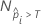# Methods and formulas for the lift chart for Random Forests® Classification

###### Note

The procedure for the points on the lift chart depends on the validation method. For a multinomial response variable, Minitab displays multiple charts that treat each class as the event in turn.

## Out-of-bag

For a given tree in the forest, a class vote for a row in the out-of-bag data is the predicted class for the row from the single tree. The predicted class for a row in out-of-bag data is the class with the highest vote across all trees in the forest. The predicted class probability for a row in the out-of-bag data is the ratio of the number of votes for the class and the total votes for the row.

For the curve for the out-of-bag data, each point on the chart represents a distinct predicted class probability. The highest event probability is the first point on the chart and appears leftmost. The other probabilities are in decreasing order.

The points on the non-cumulative lift chart do not follow from the calculation of the points on the ROC curve chart. Instead, the y-coordinate of the non-cumulative lift chart is (non-cumulative true positive rate in percent / % of population at the x-coordinate). The calculation of the true positive rate is exactly the same as for the ROC curve chart.

The x-coordinate of the chart has the following form:

whereis the number of rows where the fitted probability is greater than the threshold and N is the total number of rows. For details on the thresholds, go to Methods and Formulas for the Receiver Operating Characteristic (ROC) curve chart for Random Forests® Classification.

## Separate test set

Use the same steps as the training set case but calculate the event probabilities from the cases for the test set.

By using this site you agree to the use of cookies for analytics and personalized content.  Read our policy